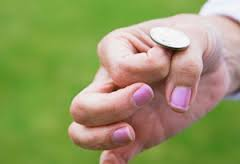# Eventually tossed a coin many times. Part-II

Probability Level 3A coin is tossed $10$ times. If the probability of getting at least six heads is $\left( \dfrac{a}{b} \right)$ where gcd(a,b)=1. Then find the value of $(a+b)$.

###### Check out the set :Just a Little Twist to fall in love with Maths and Problem Solving.
×

Problem Loading...

Note Loading...

Set Loading...